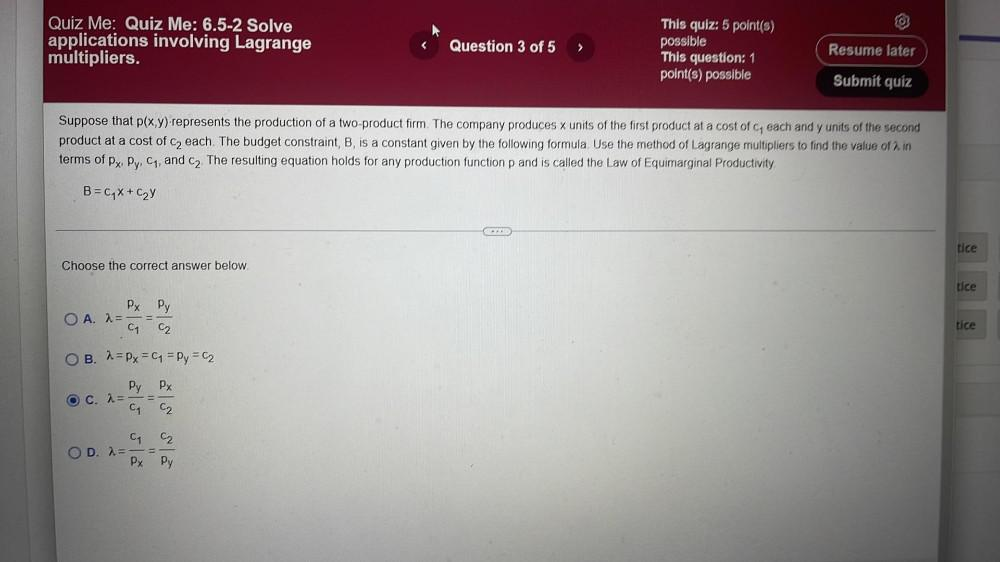Question:

# Suppose that p(x,y)-represents the production of a two-product firm. The company produces x units of the first product at a cost of c, each and y units of the second product at a cost of c₂ each. TheSuppose that p(x,y)-represents the production of a two-product firm. The company produces x units of the first product at a cost of c, each and y units of the second product at a cost of c₂ each. The budget constraint, B, is a constant given by the following formula. Use the method of Lagrange multipliers to find the value of λ in terms of px, Py, C₁, and C₂. The resulting equation holds for any production function p and is called the Law of Equimarginal Productivity. B=C₁X+C₂Y Choose the correct answer below. A. λ=px/c1=py/c2 B. λ=px=c1=p1=c2 C. λ = py/c1=px/c2 D. λ= c1/px=c2/py